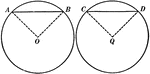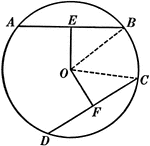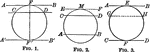### Equal Chords in Equal Circles Theorem

Illustration used to show that "In equal circles, or in the same circle, if two chords are equal, they…### Equal Chords in Equal Circles Theorem

Illustration used to show that "In equal circles, or in the same circle, if two chords are equal, they…### Circles With Parallels Intercepting Equal Arcs

Illustration of a circle with parallels intercepting equal arcs on a circumference.